# Thermodynamics Questions for Class 11, NEET and JEE

## Thermodynamics Questions

Question 1
Two thermally insulated vessels are filled with equal number of moles of air and connected by short tube with a value. If P, V and T and pressure, volume and temperature of gas in first vessel and 2P, V and T / 2 be pressure, volume and temperature of gas second vessel then find temperature and pressure of air after opening the value.

On opening of valve air at pressure P and total volume of air in both the vessels would be 2V. since number of moles of air in both the vessels is equal so. from ideal gas equation we have
for vessel
(1) PV = μ1RT
(2) 2PV = μ2RT/2
after air is mixed up
2P'V =(μ1+ μ2)RT'
Where P' and T' are final pressure and temperature
Again considering two portions of air as single system. Since the system is a thermally insulated vessel containing air so total heat transfer of combined system is zero i.e. Q = 0
Since no mechanical work is done on the system or done by the system => work done W = 0.
As no heat transferred takes place and no net amount of work has been done so there would be no change in internal energy of the combined system and would equal sum of internal energies of two partitions of air.
Ui = U1+ U2
= μ1RT/(γ-1)+μ1R(T/2)/(γ-1)
and final internal energy
Uf = (μ1+ μ2)RT'/(γ -1)
μ1RT/(γ-1) + μ1R(T/2)/(γ-1) = (μ1+ μ2)RT'/(γ -1)
or T' = (μ1+ μ2) = (2μ1+ μ2)T/2
μ1= PV/RT
μ2= 4PV/RT
=> T'[(PV/RT + 4PV/RT) = 1/2(2PV/RT + 4PV/RT)T
5T' = (1/2) 6T
T' = (3/5 )T
P' = (μ1+ μ2)RT'/2V
=5PV/RT x R/2V x 3/5 T
P' = (3/2)P

T' = (3/5 )T
P' = (3/2)P

Question 2
Calculate the heat absorbed by the system in going through one cycle for the cyclic process
shown in the Figure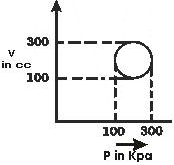Since the process is cyclic so change in internal energy would be zero.
From first law of thermodynamics
W = Q
now work done is equal to area under this cycle which is
$W = \pi R^2$
$= \pi (100 cc)(100 kpa)$
$=3.14 \times 10$
$= 31.4$ J
=> heat absorbed by system during cyclic process is 31.4 J.

Question 3
when water is boiled under a pressure of 2 atm, the heat of vaporization is $2.20 \times 10^6$ J Kg-1 and the boiling point is 120 °C. At this pressure 1 Kg of water has a volume $10_{-3}$ m3, and 1 kg of steam a volume of 0.824 m3,
(a) Compute the work done when 1 kg of steam is formed at this temperature
(b) Compute the increase in internal energy

(a) Work done in forming 1 Kg of steam at 120 °C is
$W = P dV$
= 2 x 105 x 0.824m3 x 1.01
= 1.67 x 105 J.
(b) Increase in internal energy is
$\Delta U = Q - W$
where Q = MLv
where Lv is latent heat of vaporization of water
Q = 1 kg x 2256 J / gm
= 1000 x 2256 J / gm
= 2.256 x 106 J
now $\Delta U = 2.256 \times 10^6 - .167 \times 10^6$ J
$= 2.092 \times 10^6$ J

Question 4
Figure below shows different paths. connecting state i to state f in P-V diagram along which gas can be taken Rank paths according to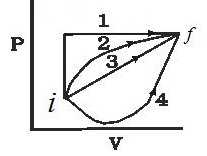(a) Change in internal energy
(b) Work done by gas
(c) The magnitude of energy transferred as heat.

(a) Change in internal energy ΔU remains same for all paths as it depends on initial and final points i and f not on the paths through which system is taken from initial point to final point
(b) Work done by gas along these paths is ranked as 1, 2, 3, 4 i.e., work done is greatest along path 1 because area under path 1 is greatest and area covered under path 4 is lowest
(c) from first law of thermodynamic
ΔU = Q - W
or Q = ΔU + W
from this magnitude of energy transferred as heat is greatest along path 1 and then subsequently on path 2,3 and 4.

Question 5
Two moles of helium (γ=5/3) are initially at a temperature of 27 °C and occupy a volume of 20L. The helium is first expanded at constant pressure until the volume has tripled, and then adiabatically until the temperature returns to its initial state. given for helium
Cp = 20.78 J/mol-k
(a) Draw diagram of process in P-V plane
(b) What is the total heat supplied in the process
(c) What is the total change in internal energy of helium.
(d) What is the total work done by the helium.
(e) What is the final volume and pressure.

(a) PV diagram of process is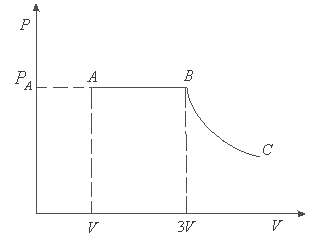(b) since the gas is expending at constant pressure so when its volume gets tripled its temperature also gets tripled.
for gas expending at constant pressure heat dQ flowing into gas is
dQ= nCpdT
Integrating both sides with limits of T going from T1 and T2
∫dQ=∫nCpdT
Q=nCp T2 - T1)
given that
Cp = 20.78 J/mol-k
n = 2 mol.
T1 = 27°C = 300 k
T2 = 3 x 300 = 900 k
= 2 x 20.78 x (900 - 300)
= 2.5 x 104 J
(c) Total change in internal energy of helium is zero as initial and final temperature of the process is same
(d) for total work done by helium calculate work done by gas in isobaric process AB and also work done by gas during adiabatic process BC.
now from ideal gas equation
PV = μRT
Initial pressure
P = μRT/V = (2 x 8.314 x 300)/(20 x 10-3)
= 2.49 x 105 N/m2
At point B
PB = PA = 2.49 x 105 N/m2
volume VB = 3VA = 60 x 10-3 m3
and TB = 900 k.
Thus work done by gas during isobaric process AB
= 2.49 x 105 (60 - 20) x 10-3
=9960 J.
Work done by gas during adiabatic process BC
= (μR/(1 - γ))(TB - TC)
= [2 x 8.314/1- (5/3)] [30-900]
;= 14940
Therefore net work done by gas
W = WAB + WBC
= 9960 J + 14940 J
= 2.49 x 104 J
TVγ-1 = Constant
TBVBγ-1 = TCVCγ-1
or, (VC / VB)γ-1 = TB/TC = 900 / 300 = 3
VC/VB = (3) 1/γ-1 = (3) 1.5
= 5.196
VC = 5.196 x VB
= 5.196 x 60 x 10-3m3
= 311.7 x 10-3m3
final pressure
PC = μRTC / VC
= 2 x 8.3 x 300 / 311.7 x 10-3
= .159 x 105 N/m3

Question 6
Temperature of a system containing 1 mole ideal gas increases by on amount ΔT = 30 K as a result of heating at constant pressure. If gas obtains an amount of heat Q= 3.2 KJ. then calculate
(a) work done by the gas
(b) change in its internal energy
(c) value of γ= Cp / Cv

(a) Work done when system undergoes isobaric process is
W = PdV
from ideal gas equation
PV = μRT
here μ = 1
=> PdV = RμT
or W = RΔT
, = 8.314 x 80
= .665 KJ
(b) from first law of thermodynamics
ΔU = Q- W = Q- RΔT
given that Q= 3.2 KJ
ΔU = 3.2 KJ - .665 KJ
= 2.536 KJ
(C) Again the know that
ΔU = μCvΔT
= RΔT / γ-1
γ-1 = RΔT / ΔU
or γ= (RΔTT / ΔU) +1
= (.665 KJ x 8.314/ 2.535KJ) +1
= 1.26

Question 7
Two moles of a certain ideal gas at a temperature T0 = 300K were cooled isochorically so that the gas pressure reduced two times. Then as a result of the isobaric process the gas expanded till its temperature get back to the initial value.
(a) What would be total change in internal energy.
(b) Total work done by the system.
(c) Find the total amount of heat absorbed by gas in this process

(a) Change in internal energy ΔU = 0 for the whole process
(b) during Isochoric process work done would be equal to zero = W1 = 0
now work done during isobaric process is
W = PΔV
= μRΔT
At the end of Isochoric process temperature of the gas can be calculated as follows
In the beginning of Isochoric process ideal gas equation is
PV=nRT0 (1)
During Isochoric process volume remains constant and at the end of the process pressure becomes P/2 and temperature becomes T'
Thus at the end of process ideal gas equation becomes
PV=nRT' (2)
From 1 and 2 T'=T0/2
Now since temperature is increasing from T0/2 to T0
W=μR(T0 -(T0 /2))
(c) total heat absorbed is given by ΔU = Q-W
from first law of thermodynamics
Q= W
total heat absorbed
Q= μRT0 (1-1/n)
= 2 x 8.314 x 300 x (1-1 / 2)
= 2494.25
= 2.5 KJ

Question 8
An ideal gas where adiabatic exponent is equal to γ, is expanded so that the amount of heat transferred to the gas is equal to the decrease of internal energy find
(a) molor heat capacity of gas in this process
(b) equation of process in variables T, V
(c) Work performed by one mole of gas when its volume increases by η time if T0 is the initial temperature of the gas.

(a) As given in the question
$Q= -\Delta U$
so $dQ= -dU= - \mu C_v dT$
$= \frac {\mu R}{\gamma -1} dT$
now molar heat capacity
$C = (\frac {1}{\mu}) (\frac {dQ}{dT})$
$= - \frac {R }{ \gamma -1}$
(b) From first law of thermodynamics we have
$dQ= dU + dW$
but here : dQ= -dU
$- dU= dU+ dW$
or $- 2 dU = dW$
or $-2 \mu C_v dT = P dV$
or $(-2\ mu \frac {R }{ \gamma -1} dT = (\frac {\mu RT }{ V}) dV$
$(\frac {dT}{ T}) + (\frac {\gamma -1 }{ 2 V})(\frac {dV}{V})=0$
On integrating me get
$TV^{\frac {\gamma -1}{2}} = constant$
which is the required equation
(c) As found earlier work done
$dW = - 2dU$
or $W = - 2 (U_2 - U_1 )$
$= -2 C_vT_0((\frac {T }{ T_0}) - 1)$
= 2 CV T0 (1 - (T / T0))
Now as we know that
TV(γ-1)/2 = constant = T0V0(γ-1)/2
(T / T0) = (V0 / V) (γ-1)/2 = 1-(1 / η(γ-1)/2)
So, W = 2 Cv T0 (1 - T / T0)
=2 CV T0 [1 - 1 / (η)(γ-1)/2]
= (2RT0 / γ-1) [1 - 1 / (η)(γ-1)/2]

Question 9
An ideal gas is taken from an initial state i to a final state f in such a way that the ratio of the pressure to the absolute temperature remains constant. What will be the work done by the gas.

Given that
P / T = const.
from ideal gas equation
P / T = μR / V = constant
V = μRT / P = constant
Since volume remains constant in this process =>V = 0
or W = pdV = 0
Work done during the process is zero.

Question 10
Consider the cyclic process ABCA, shown in the Figure, performed on a sample of 2.0 mole of an ideal gas. A total of 1200 J of heat is withdrawn from the sample as the process. Find the work done by the gas during the part BC.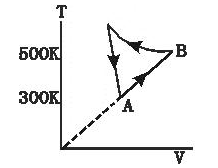Given that number of moles of gas is μ= 2.0 mole and heat rejected Q= 1200 J.
given process is cyclic so dU= 0
from first law of thermodynamic
dQ= dW
during CA volume remains constant
WCA = 0
along AB
T ∝ V
=> T /V = const.
now from ideal gas eqn.
PV = μRT
P=μRT /V=constant
WAB = P(V2 - V1)
= μRT2- μRT1
= 2 x 8.3 x (500 - 300)
= 3320 J
now total work done
W = WAB + WBC
W = 3320 + WBC
from first law of thermodynamics
Q= W
AS heat is being taken of
-Q = W
- 1200 J = 3320 J + WBC or WBC = - 4520 J

Question 11
Consider a cylindrical tube of volume V with adiabatic walls containing an ideal gas. The internal energy of this ideal gas is given by 1.5 μRT. The tube is divided into two equal parts by a fixed diathenic wall. Initially, the pressure and the temperature are P1, T1 on the left side and P2, T2 right. The system is left for sufficient time so that the temperature becomes equal on the two sides.
(a) How much work has been done on the left part.
(b) Find the final temperature on the two sides.
(c) Find the final pressure on the two sides.

(a) W = 0 since vessel has fixed volume and no heat is exchanged between system and surrounding total internal energy of system will remain constant.

(b) Total energy of system before and after the process will remain some now before process internal energy of system is
Ui= 1.5 μ1 RT1 + 1.5 μ2 RT2
and after the process
Uf= 1.5 (μ1 + μ2) RT
where T is the final equilibrium temperature
Now Ui=Uf
1.5 μ1 RT1 + 1.5 μ2 RT2= 1.5 (μ1 + μ2) RT
or T = (μ1T1 + μ2T2) / (μ1 + μ2
) from ideal gas equation
μ= PV / RT
=> μ1 = P1V1 / RT1
=> μ2 =P2V2 / RT2
So
T = (P1 + P2) T1T2 / (P1T2 + P2T1)
or T = (P1 + P2) T1T2
where λ = P1T2 + P2T1

c) If P1' and P2' are pressures on left and right sides respectively then
P1V1/ RT1 =P1'V1 / RT
=>
P1' = P1T / T1
putting value for T
= P1 (P1 + P2) T1T2 /λ T1
or P1' = T2P1 (P1 + P2) T1T2
similarly
P2' = P2T / T2
P2' = T1P2 (P1 + P2) T1T2

Question 12
1 moles of an ideal gas whose adiabatic exponent equals y undergoes a process in which gas pressure relates to the temperature as
P=aT2 where a is constant.
If the temperature get an increment of ΔT,find following
a. Change in Internal energy
b. Work performed by the gas
c. Molar heat capacity of the gas in the process
d. find the amount heat supplied

a. Change in internal energy = CvΔT=RΔT/(y-1)
b. work done by the gas
given that
P=aT2
Now we know that PV=RT
so, P=a(PV/R)2
PV2=R2/a
PV2=Constant
Now we know that
for PVy=Constant
Work done is given as=RΔT/(1-y)
Similarly for PVn=Constant
Work done =RΔT/(1-n)
Therefore work done in this case
W=RΔT/(1-2)
=-RΔT

c. Molar heat capacity is given by

$C= \frac {dQ}{dT}$
$C= \frac {C_v dT + P dV}{dT}$

C= Cv + P(dV/dT)
=Cv + (RT/V)(dV/dT)
Now P=aT2
RT=aVT2 as PV=RT
VT=constant
TdV + VdT=0
dV/dT=-V/T
So C= Cv + (RT/V)(-V/T)
=Cv -R
=[{R/(y-1)} -R]

d. Q= ΔU +W
= [{RΔT/(y-1)} -RΔT]
=ΔT[R/(y-1) -R]

Question 13
Two vessels A & B of equal volumes V0 are connected by the narrow tube which can be closed by the valve. The vessels are fitted with the piston which can be moved to change the volumes.Initially the valve is open and vessel contains ideal gas whose adiabatic exponents is Y at atmosphere pressure P0 and atmosphere temperature T0.The walls of the vessel A are adiabatic while the walls of the vessels B are diathermic. The valve is now closed and piston is slowly moved to triple the original volume.
a. Find the temperature and pressure in the two vessels.
b The valve is now open for sufficient time so that gases acquire a common temperature and pressure. Find the new values of pressure and temperature

a. for Vessel A ,walls are adiabatic
so PVγ=constant

P0V0γ= P(3V0)γ
P= P0/3γ

Also
TVγ-1=constant

T= T0/3γ-1

For vessel B ,wall are diathermic, so final temperature is going to be T0 only

So P0V0=P* 3V0
P=P0/3

b. Now when the valve is opened again, final temperature will be again T0

Also
Initially P0V0=nRT0
finally
P*3V0=nRT0

therefore
P=P0/3

Question 14
1 mole of an ideal gas whose adiabatic exponents is γ is enclosed in the vertical adiabatic vessel fitted with moving frictionless piston whose weight is W and cross-sectional area is A.The atmospheric pressure is P0.Initial volume is V0.Heat is supplied to the gas to double its volume.
a. Find the initial and final state of the gas
b. Find the work done by the gas
C. Find the change in internal energy

a.
Initial State
Initial Pressure = [P0 + (W/A)]
Initial volume = V0
Initial temperature from PV=RT
=[P0 + (W/A)]V0/R
Final state
Pressure remain constant so

Final Pressure = [P0 + (W/A)]
Final volume = 2V0
Final temperature from PV=RT
=2[P0 + (W/A)]V0/R

b. Work done by the gas
= ∫PdV

Since pressure remains constant
=[P0 + (W/A)]V0

c. Change in internal energy is given by

= CVΔT
= [{R/(γ-1)})[P0 + (W/A)]V0/R
= [P0 + (W/A)][V0/(γ-1)]

d Heat supplied
Q= ΔU +W
= [P0 + (W/A)][V0/(γ-1)] + [P0 + (W/A)]V0
=[P0 + (W/A)][V0y/(γ-1)]

Question 15
Two samples A and B whose adiabatic exponents is γ are initially kept in the same state (P0,V0,T0).Now sample A is expanded such that volume becomes 2V0 and Q=0 for the process. Sample B is expanded such that volume becomes 2V0 and ΔU=0 for the process
a. Find the ratio of the final pressure of the two samples
b. Find the ratio of the final temperature of the two samples
c. Find the ratio of the work done by the two samples

a. For sample A
P0V0γ= PA(2V0)γ
PA=P0/2γ
For sample B
Since ΔU=0,therefore Isothermal
So P0V0=PB 2V0
PB=P0/2

So PA : PB = 1 : 2γ-1

b. For sample A
T0V0γ-1=TA(2V0)γ-1
TA = T0/2γ-1

for sample B
TB = T0

So TA : TB = 1 : 2γ-1

c. Work done by the Sample A

$W=\frac {P_iV_i -P_fV_f}{\gamma -1}$
$=\frac {P_0V_0 - (\frac {P_0}{2^{\gamma} }) \times 2V_0}{\gamma -1}$
$=\frac {P_0V_0 (2^{\gamma -1} -1)}{2^{\gamma -1}(\gamma -1)}$
Work done by the Sample B
WB= RT0ln2
= P0V0ln2

Now ratio
WA : WB= 1-21-y : (y-1)ln2

Question 16
One mole of an ideal monatomic gas is taken round the cyclic process ABCDA as shown in figure.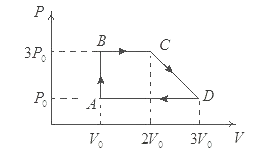a. Work done by the gas
b Heat absorbed by the gas in AB and BC
c. Heat in process CD
d. Find the temperature at C and D
e. Maximum temperature attained by the gas during the cycle
f. Net change in the internal energy and the heat

For monatomic gas
Cp=5R/2
Cv=3R/2

a Work done by the gas = Area enclosed by the curve ABCDA
=3P0V0

b. Heat absorbed the gas in AB
QAB=Cv(TB-TA)
=3R/2(3P0V0/R -P0V0/R)
=3P0V0
Heat absorbed the gas in BC
QBC=CP(TC-TB)
=5R/2(6P0V0/R - 3P0V0/R)
=15P0V0/2

c. Heat rejected in DA
QDA=CP(TA-TD)
=-5P0V0

Now for the cycle process
QAB+QBC+QCD+QDA=W
So
QCD= -5P0V0/2
d. from diagram and PV=RT

TC=6P0V0/R
TD=3P0V0/R

e. Max temperature will be on the slope CD

Equation of Slope CD as Coordinated system
P=-(2P0/V0)V + 7P0
Now PV=RT

So
RT= -(2P0/V0)V2 + 7P0

For max
dT/dV should be zero

So RdT/dV=-4P0V/V0 +7
V=7V0/4
Tmax= 49P0V0/4R

f. ΔU=0
Net heat=3P0V0

Question 17
An ice cube of mass .1kg at 0 °C is placed in an isolated container which is at 227° C. The specific heat of the container varies with temperature according to the empirical formula
S=A + BT
where A is 100cal/kg-K and B is 2x10-2 cal/kg-K2
if the final temperature of the container is 27°C.Determine the mass of the container.
Specific heat of water is 103 cal/kg-K
Latent heat of fusion of water is 8x104 cal/kg

Let m be the mass of the container. Since specific heat varies with temperature, we will have to integrate to total heat lost from container.
dQ=msdT
dQ=m(A+BT)dT
Integrating for the upper and inner limit 300 and 500 respectively
Q= ∫m(A+BT)dT
Q=m[AT +BT2/2]
=-21600m

now heat gained by the ice
Q= heat given to 0° (ice) to 0°(water) + heat required to raise the temperature from o to 27°C
Q=mL + mcΔT
=.1 * 80000 + .1*10-3*27
=10700 cal
Now heat gained =Heat lost
So
21600m=10700
m=.495kg

Question 18
A gaseous mixture is such that
 Gas No of moles Adiabatic coefficient A 1 1.67 B 2 1.4

The gaseous mixture is taken through an adiabatic process where temperature of the mixture drops by ΔT.
a. Find the work done by the mixture
b. If m1 and m2 are the relative molecular mass of the gas A and B respectively. Find the speed of sound in the mixture at Temperature T0

a.Let Cp ,Cv and ym are the heat capacities and adiabatic coefficient of the mixture.
then ym=Cp/Cv

Now Cp=nACpA+nBCpB/nA+nB
Also Cv=nACvA+nBCvB/nA+nB

So ym=nACpA+nBCpB/nACvA+nBCvB

Substituting the values
ym =1*5/2R + 2*7/2R/1*3/2R +2*5/2R
=19/13

Work done by the mixture is given as
=nRΔT/y-1
=39RΔT/6
b. Molar mass of the mixture will be =m1+2m2

Speed of sound=√yRT/M

Question 19
A diatomic gas whose adiabatic coefficient is γ=1.4 is taken through XYZAX cycle.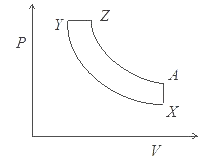Y->Z Isobaric expansion
A->X Isochoric process
find the following
1. Molar heat capacities in each process
2. if the volume ratio is VX/VY=16 and VZ/VY=2 and TY=636° C
a. find the heat absorbed in the process Y->Z
b. find the net work done by the gas in the cycle
c. find the ratio of pressure PY/PX and PZ/PA

n=1
γ=1.4

1. Molar heat capacity of the gas in the Process X->Y (Adiabatic)=0 as Heat is zero
Molar heat capacity of the gas in the Process Y->Z (pressure constant)=Cp =7/2R
Molar heat capacity of the gas in the Process Z->A (Adiabatic)=0 as Heat is zero
Molar heat capacity of the gas in the Process A->X (Volume constant)=Cv =5/2R
2. VX/VY=16
TXVXγ-1=TYVYγ-1
TX= TY(VY/VX)γ-1
=909 (1/16).4
=300K
Now Y->Z ,According to Charles law
VZ/TZ=VY/TY
So TZ= 909*2=1818 K

Heat absorbed in Y->Z>
=nCp(TZ-TY)
=.3182R

WXY= -nR(TY-TX)/γ-1
=-5R/2* 609
WYZ=nR(TZ-TY)
=909R
WZA= -nR(TZ-TA)/γ-1

Now we know that VX/VY=16 and VZ/VY=2
as VX=VA
So VZ/VA=1/8

TZVZγ-1=TAVAγ-1
TA=TZ(VZ/VA)γ-1
=791K

So
WZA =5R/2 * 1027

Also WAX=0 as volume constant

Then
WNET=909R + 5R/2(1027-609)
=1954R

PXVXγ=PYVYγ
PY/PX= 161.4

Similarly

PZVZγ=PZVZγ
PZ/PA= 81.4

Question 20
Consider one mole of perfect gas in a cylinder of unit cross section with a piston attached in below figure. A spring (spring constant k) is attached (unstrectched length L ) to the piston and to the bottom of the cylinder. Initially the spring is unstrectched and the gas is in equilibrium. A certain amount of heat Q is supplied to the gas causing an increase of volume from $V_0$ to $V_1$.
(a) What is the initial pressure of the system?
(b) What is the final pressure of the system?
(c) Using the first law of thermodynamics, write down a relation between Q, $P_a$, V, $V_0$ and k.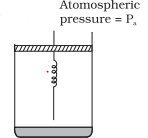(a): It is considered that piston is mass less and piston is balanced by atmospheric pressure ($P_a$). So the initial pressure of system inside the cylinder = $P_a$.
(b) On supply heat Q. Volume of gas increases from $V_0$ to $V_1$ and spring stretched also.
$\Delta V = V_1 - V_0$
If displacement of piston is x then volume increase in cylinder
$\Delta V= \text {Area of base} \times {height} = A \times x$
$A \times x = V_1 - V_0$
$x = \frac {V_1 -V_0}{A}$
$x=V_1 -V_0$
As A=1
Force exerted by spring $F_S = K(V_1 -V_0 )$
So final total pressure on gas $P_f = P_a + K(V_1 - V_0)$
(c) By Ist law of thermodynamics
$dQ = dU + dW$
$dU = C_V(T - T_0)$
$T = \frac {PV}{R} = \frac {[P_a + K(V_1 - V_0)]V_1}{R}$
Now
W.D. by gas = p. dV + increase in PE of spring
$dW = P_a(V_1 -V_0) + \frac {1}{2} K(V_1 -V_0)^2$
Now $dQ = dU + dW$
So,
$Q= C_V(T - T_0) + P_a(V_1 -V_0) + \frac {1}{2} K(V_1 -V_0)^2$
Where $T= \frac {[P_a + K(V_1 - V_0)]V_1}{R}$
It is required relation.